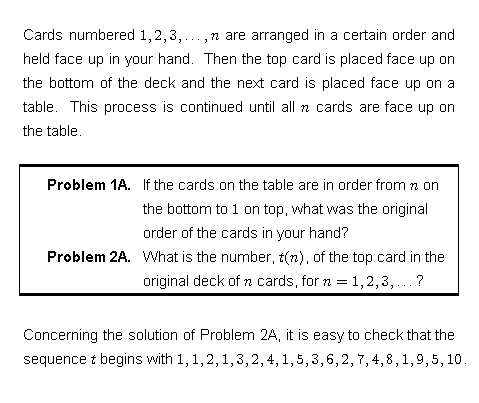# FRACTAL SEQUENCES

 Probably, fractal sequences are first defined in the following article: C. Kimberling, "Numeration systems and fractal sequences," Acta Arithmetica 73 (1995) 103-117. Fractal sequences have in common with the more familiar geometric fractals the property of self-containment. An example of a fractal sequence is 1, 1, 2, 1, 3, 2, 4, 1, 5, 3, 6, 2, 7, 4, 8, 1, 9, 5, 10, 3, 11, 6, 12, 2, 13, 7, 14, 4, 15, 8, . . . If you delete the first occurrence of each positive integer, you'll see that the remaining sequence is the same as the original. (So, if you do it again and again, you always get the same sequence.) This particular sequence, by the way, solves an interesting card-sorting problem posed by my co-author in the paper C. Kimberling and Harris S. Shultz, "Card sorting by dispersions and fractal sequences," Ars Combinatoria 53 (1999) 209-218.Another example of a fractal sequence is the signature sequence of a positive irrational number, R: arrange the set of all numbers i + j R, where i and j are positive integers, in order:

i(1) + j(1) R < i(2) + j(2) R < i(3) + j(3) R < . . .

Then i(1), i(2), i(3), . . . defines the signature of R. For example, the signature of the square root of 2 starts with

1, 2, 1, 3, 2, 1, 4, 3, 2, 5, 1, 4, 3, 6, 2, 5, 1, 4, 7, 3, 6, 2, 5, 8, 1, 4, 7, 3, 6, 9, 2, 5, 8, . . .

More on signatures and other fractal sequences is proved in

C. Kimberling, "Fractal sequences and interspersions," Ars Combinatoria 45 (1997) 157-168.

Fractal sequences are closely related to interspersions and dispersions.

Interspersions and Dispersions
Clark Kimberling Home Page